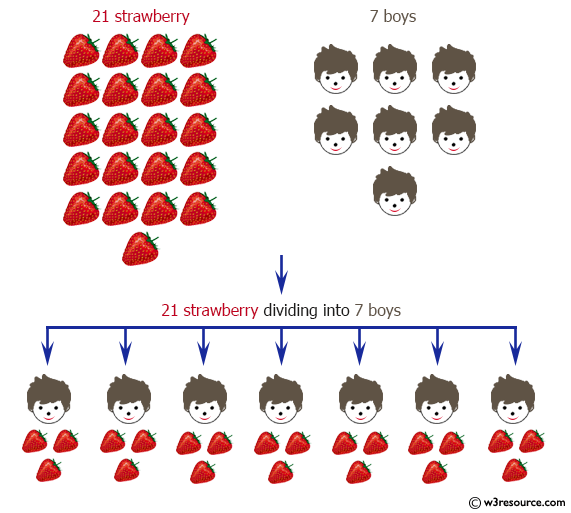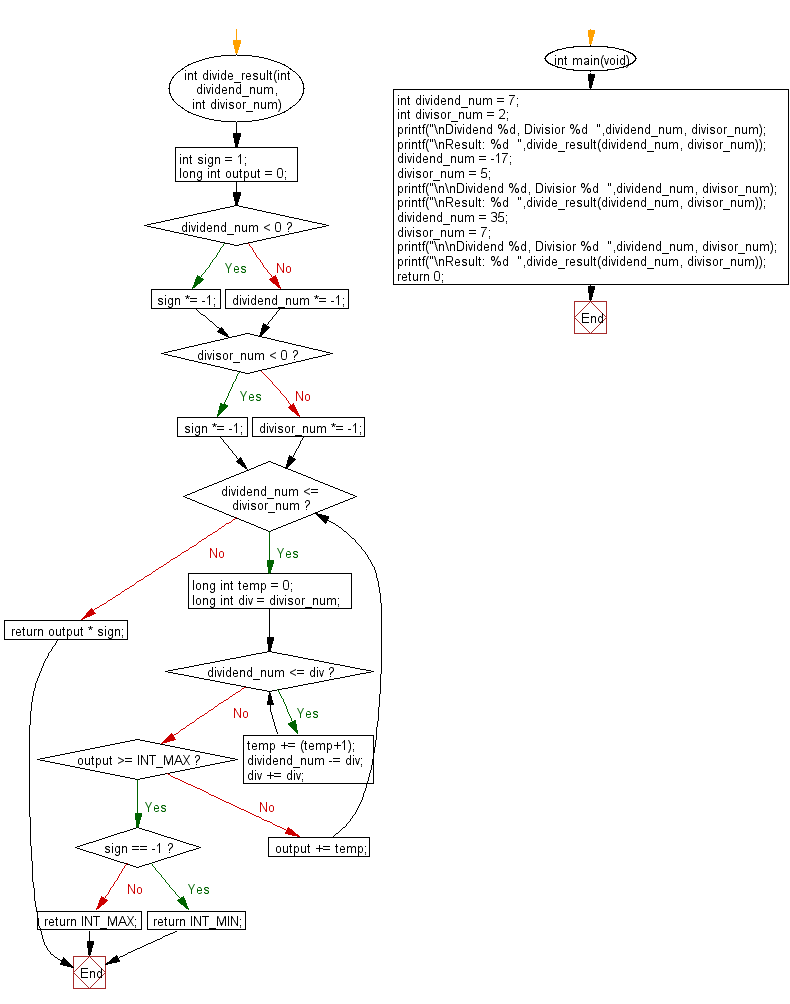﻿ C Program: Divide without multiply, division, mod

# C Exercises: Divide two integers without using multiplication, division and mod operator

## C Programming Mathematics: Exercise-3 with Solution

Write a C program to divide two integers (dividend and divisor) without using the multiplication, division and mod operator.

Example:
Input:
dividend_num = 7
divisor_num = 2

dividend_num = -17
divisor_num = 5

dividend_num = 35
divisor_num = 7
Output:
Result: 3
Result: -3
Result: 5

Pictorial Presentation:Sample Solution:

C Code:

``````#include <stdio.h>
#include <limits.h>
int divide_result(int dividend_num, int divisor_num){
int sign = 1;
long int output = 0;
if (dividend_num < 0) {
sign *= -1;

} else {
dividend_num *= -1;
}
if (divisor_num < 0) {
sign *= -1;

} else {
divisor_num *= -1;
}
while (dividend_num <= divisor_num) {
long int temp = 0;
long int div = divisor_num;
while (dividend_num <= div) {
temp += (temp+1);
dividend_num -= div;
div += div;
}
if (output >= INT_MAX) {
if (sign == -1) {
return INT_MIN;
} else {
return INT_MAX;
}
}
output += temp;
}
return output * sign;
}
int main(void)
{
int dividend_num = 7;
int divisor_num = 2;
printf("\nDividend %d, Divisior %d  ",dividend_num, divisor_num);
printf("\nResult: %d  ",divide_result(dividend_num, divisor_num));
dividend_num = -17;
divisor_num = 5;
printf("\n\nDividend %d, Divisior %d  ",dividend_num, divisor_num);
printf("\nResult: %d  ",divide_result(dividend_num, divisor_num));
dividend_num = 35;
divisor_num = 7;
printf("\n\nDividend %d, Divisior %d  ",dividend_num, divisor_num);
printf("\nResult: %d  ",divide_result(dividend_num, divisor_num));
return 0;
}
```
```

Sample Output:

```Dividend 7, Divisior 2
Result: 3

Dividend -17, Divisior 5
Result: -3

Dividend 35, Divisior 7
Result: 5
```

Flowchart:C Programming Code Editor:

Improve this sample solution and post your code through Disqus.

What is the difficulty level of this exercise?

Test your Programming skills with w3resource's quiz.

﻿